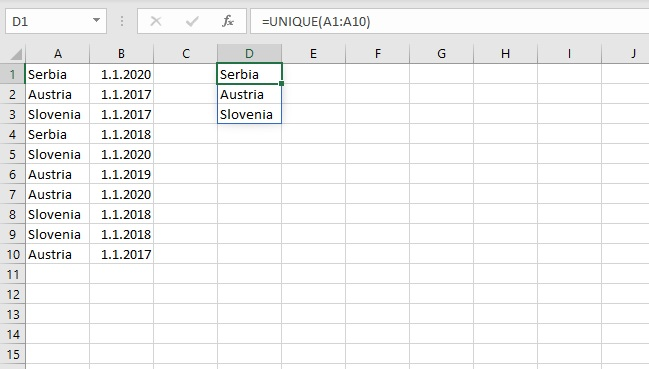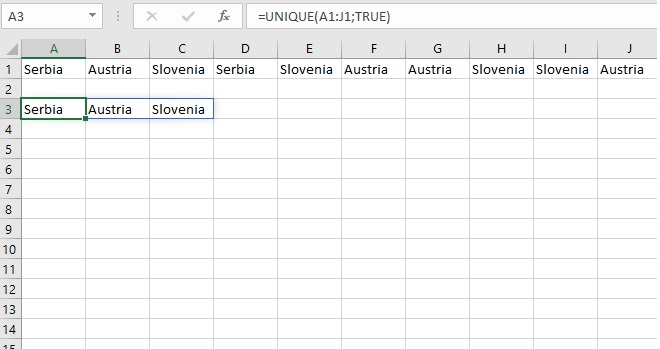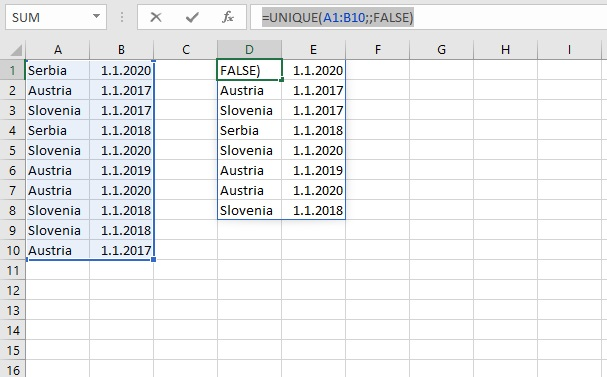# UNIQUE function

Sometimes you have repeating values ​​in the column and you want to extract only their unique instances. The Remove Duplicates option is used to do this, and the UNIQUE is a new Excel function that has been built to perform the same thing. It generates a dynamic array with unique values, whether it contains text, numbers, dates …

The syntax of the UNIQUE function is:

UNIQUE (<range>,[<by column>],[<exactly once>])

where range represents the spreadsheet area from which we retrieve values. The optional “by column” argument is used when the data, instead of rows, is retrieved from columns. The default value is FALSE, and if the data is in columns it is necessary to set the value TRUE. The last, also optional, argument is used if we want the function to return values ​​that are repeated exactly once (TRUE), while default action assumes that all values ​​are displayed (FALSE).

If we enter the formula:

=UNIQUE(A1:A10)as a result, three unique countries are extracted from the list in column A.

If the data were, in some cases, in rows, we would write the formula:

=UNIQUE(A1: J1,TRUE)If we want to extract unique repetitions for two columns, we need to set the third parameter as FALSE. E.g.:

=UNIQUE(A1: B10,,FALSE)